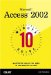# Using the Total Row in a Query

 Team-Fly10 Minute Guide to Microsoft Access 2002 By Joe Habraken Table of Contents Lesson 18.  Creating Queries from Scratch

Using the Total Row in a Query

You can also do calculations in a query, such as totaling numeric information in a particular field or taking the average of numeric information found in a particular field in the query. To add calculations to a query, you must add the Total row to the Query Design grid.

After the Total row is available in the query grid, different calculations can be chosen from a drop-down list in any of the fields that you have chosen for the query. For example, you can sum (total) the numeric information in a field, calculate the average, and even do more intense statistical analysis with formulas such as minimum, maximum, and standard deviation.

To add a calculation to a field in the query grid, follow these steps:

1. In Query Design view, click the Totals button on the Query Design toolbar. The Total row is added to the Query Design grid (just below the Table row).

2. Click in the Total row for a field in the Query Design grid that contains numerical information. A drop-down arrow appears.

3. Click the drop-down arrow (see Figure 18.5) to select the formula you want to place in the field's Total box. The following are some of the more commonly used formula expressions:

• Sum Totals the values found in the field.

• Avg Calculates the average for the values found in the field.

• Min Displays the lowest value (the minimum) found in the field.

• Max Displays the highest value (the maximum) found in the field.

• Count Calculates the number of entries in the field; it actually "counts" the entries.

• StDev Calculates the standard deviation for the values in the field. The standard deviation calculates how widely values in the field differ from the field's average value.

4. Repeat steps 2 and 3 to place formulas into other field columns .

When you use the Total row, you can summarize the information in a particular field mathematically when you run the query. For example, you might want to total the number of orders for a particular product, so you would use the sum formula provided by the Total drop-down list.

##### Figure 18.5. Calculations added to the Total row are chosen from a drop-down list.Team-FlyTop10 Minute Guide to Microsoft Access 2002
ISBN: 0789726319
EAN: 2147483647
Year: 2000
Pages: 160
Authors: Joe Habraken